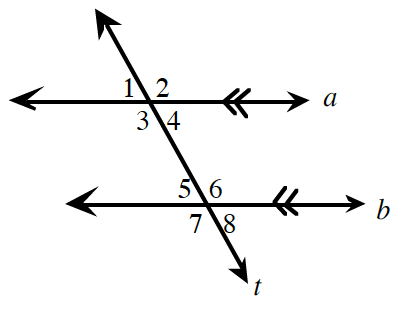### Home > CCAA8 > Chapter 9 Unit 3 > Lesson CC3: 9.2.2 > Problem9-78

9-78.

Solve for $x$. Each part is a separate problem.

1. If $m∠1 = 3x - 18º$ and $m∠5 = 2x + 12^\circ$, find $x$.

Angles 1 and 5 are corresponding angles. Since lines $a$ and $b$ are parallel, the angles are equal.

$x = 30º$

2. If $m∠3 = 4x - 27º$ and $m∠6 = x + 39º$, find $x$.

Angles 3 and 6 are alternate interior angles. Since lines $a$ and $b$ are parallel, the angles are equal.

3. If $m∠4 = 49º$ and $m∠6 = 5x + 41º$, find $x$.

Angles 4 and 6 are supplementary because the lines cut by the transversal are parallel.

$49 + (5x + 41) = 180$